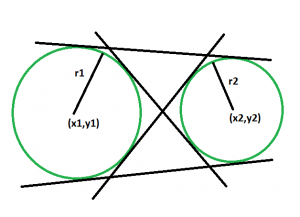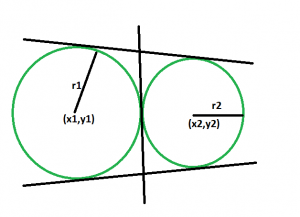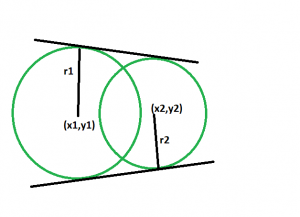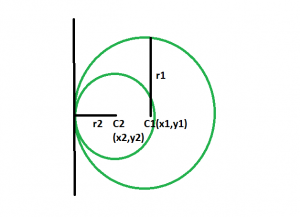Open in App
Not now

# Number of common tangents between two circles if their centers and radius is given

• Last Updated : 25 Mar, 2023

Given two circles with a given radius and centers. The task is to find the number of common tangents between these circles.
Examples:

```Input: x1 = -10, y1 = 8, x2 = 14, y2 = -24, r1 = 30, r2 = 10
Output: 3

Input: x1 = 40, y1 = 8, x2 = 14, y2 = 54, r1 = 39, r2 = 51
Output: 2```

Approach

• First of all we will check whether the circles touch each other externally, intersect each other or do not touch each other at all.(Please refer here)• Then if the circles do not touch each other externally, then obviously they will have 4 common tangents, two direct and two transverse.• If the circles touch each other externally, then they will have 3 common tangents, two direct and one transverse.
The tangent in between can be thought of as the transverse tangents coinciding together.• If the circles intersect each other, then they will have 2 common tangents, both of them will be direct.
•• If one circle is inside another circle, then they will have only one common tangent

Algorithm:

Step 1: Create the “circle” function, which has six inputs: x1, y1, x2, y2, r1, and r2.
Step 2: Use the following formula to determine the separation between the centers of the two circles: (x1 – x2)^2 + (y1 – y2)^2. Use the formula                    (r1 + r2)^2 to determine the total of the two circles’ radii.
Step 3: To ascertain the relationship between the two circles, compare the computed distance and the sum of radii:                                                                  a. If the distance is equal to the sum of radii, return 1 as there is only one common tangent.
b. If the distance is greater than the sum of radii, return -1 as there are four common tangents.
c. If the distance is less than the sum of radii, return 0 as there are two common tangents.

Below is the implementation of the above approach:

## C++

 `// C++ program to find` `// the number of common tangents` `// between the two circles`   `#include ` `using` `namespace` `std;`   `int` `circle(``int` `x1, ``int` `y1, ``int` `x2,` `        ``int` `y2, ``int` `r1, ``int` `r2)` `{`   `    ``int` `distSq = (x1 - x2) * (x1 - x2)` `                ``+ (y1 - y2) * (y1 - y2);`   `    ``int` `radSumSq = (r1 + r2) * (r1 + r2);`   `    ``if` `(distSq == radSumSq)` `        ``return` `1;` `    ``else` `if` `(distSq > radSumSq)` `        ``return` `-1;` `    ``else` `        ``return` `0;` `}`   `// Driver code` `int` `main()` `{` `    ``int` `x1 = -10, y1 = 8;` `    ``int` `x2 = 14, y2 = -24;` `    ``int` `r1 = 30, r2 = 10;` `    ``int` `t = circle(x1, y1, x2,` `                ``y2, r1, r2);` `    ``if` `(t == 1)` `        ``cout << ``"There are 3 common tangents"` `            ``<< ``" between the circles."``;` `    ``else` `if` `(t < 0)` `        ``cout << ``"There are 4 common tangents"` `            ``<< ``" between the circles."``;` `    ``else` `        ``cout << ``"There are 2 common tangents"` `            ``<< ``" between the circles."``;`   `    ``return` `0;` `}`

## Java

 `// Java program to find` `// the number of common tangents` `// between the two circles` `import` `java.io.*;`   `class` `GFG` `{` `    `  `static` `int` `circle(``int` `x1, ``int` `y1, ``int` `x2,` `        ``int` `y2, ``int` `r1, ``int` `r2)` `{`   `    ``int` `distSq = (x1 - x2) * (x1 - x2)` `                ``+ (y1 - y2) * (y1 - y2);`   `    ``int` `radSumSq = (r1 + r2) * (r1 + r2);`   `    ``if` `(distSq == radSumSq)` `        ``return` `1``;` `    ``else` `if` `(distSq > radSumSq)` `        ``return` `-``1``;` `    ``else` `        ``return` `0``;` `}`   `// Driver code` `public` `static` `void` `main (String[] args)` `{`   `    ``int` `x1 = -``10``, y1 = ``8``;` `    ``int` `x2 = ``14``, y2 = -``24``;` `    ``int` `r1 = ``30``, r2 = ``10``;` `    ``int` `t = circle(x1, y1, x2,` `                ``y2, r1, r2);` `    ``if` `(t == ``1``)` `        ``System.out.println (``"There are 3 common tangents"``+` `                    ``" between the circles."``);` `    ``else` `if` `(t < ``0``)` `        ``System.out.println (``"There are 4 common tangents"``+` `            ``" between the circles."``);` `    ``else` `        ``System.out.println (``"There are 2 common tangents"` `+` `                ``" between the circles."``);`   `    `  `}` `}`   `// This code is contributed by ajit.`

## Python3

 `# Python3 program to find` `# the number of common tangents` `# between the two circles`   `def` `circle(x1, y1, x2,y2, r1, r2):`     `    ``distSq ``=` `(x1 ``-` `x2) ``*` `(x1 ``-` `x2)``+` `(y1 ``-` `y2) ``*` `(y1 ``-` `y2)`   `    ``radSumSq ``=` `(r1 ``+` `r2) ``*` `(r1 ``+` `r2)`   `    ``if` `(distSq ``=``=` `radSumSq):` `        ``return` `1` `    ``elif` `(distSq > radSumSq):` `        ``return` `-``1` `    ``else``:` `        ``return` `0`     `# Driver code` `x1,y1 ``=` `-``10``,``8``;` `x2,y2 ``=` `14``,``-``24``;` `r1,r2 ``=` `30``,``10``;`   `t ``=` `circle(x1, y1, x2,y2, r1, r2);`   `if` `(t ``=``=` `1``):` `    ``print``(``"There are 3 common tangents between the circles."``)` `elif` `(t < ``0``):` `    ``print``(``"There are 4 common tangents between the circles."``)` `else``:` `    ``print``(``"There are 2 common tangents between the circles."``)`   `# This code is contributed by mohit kumar 29`

## C#

 `// C# program to find` `// the number of common tangents` `// between the two circles` `using` `System;`   `class` `GFG` `{` `    `  `static` `int` `circle(``int` `x1, ``int` `y1, ``int` `x2,` `        ``int` `y2, ``int` `r1, ``int` `r2)` `{`   `    ``int` `distSq = (x1 - x2) * (x1 - x2)` `                ``+ (y1 - y2) * (y1 - y2);`   `    ``int` `radSumSq = (r1 + r2) * (r1 + r2);`   `    ``if` `(distSq == radSumSq)` `        ``return` `1;` `    ``else` `if` `(distSq > radSumSq)` `        ``return` `-1;` `    ``else` `        ``return` `0;` `}`   `// Driver code` `public` `static` `void` `Main (String []args)` `{`   `    ``int` `x1 = -10, y1 = 8;` `    ``int` `x2 = 14, y2 = -24;` `    ``int` `r1 = 30, r2 = 10;` `    ``int` `t = circle(x1, y1, x2,` `                ``y2, r1, r2);` `    ``if` `(t == 1)` `        ``Console.WriteLine (``"There are 3 common tangents"``+` `                    ``" between the circles."``);` `    ``else` `if` `(t < 0)` `        ``Console.WriteLine (``"There are 4 common tangents"``+` `            ``" between the circles."``);` `    ``else` `        ``Console.WriteLine (``"There are 2 common tangents"` `+` `                ``" between the circles."``);`   `}` `}`   `// This code is contributed by Arnab Kundu`

## PHP

 ` ``\$radSumSq``)` `        ``return` `-1;` `    ``else` `        ``return` `0;` `}`   `    ``// Driver code` `    ``\$x1` `= -10; ``\$y1` `= 8;` `    ``\$x2` `= 14; ``\$y2` `= -24;` `    ``\$r1` `= 30; ``\$r2` `= 10;` `    ``\$t` `= circle(``\$x1``, ``\$y1``, ``\$x2``,` `                ``\$y2``, ``\$r1``, ``\$r2``);` `    ``if` `(``\$t` `== 1)` `        ``echo` `"There are 3 common tangents"` `                ``,``" between the circles."``;` `    ``else` `if` `(``\$t` `< 0)` `        ``echo` `"There are 4 common tangents"` `            ``, ``" between the circles."``;` `    ``else` `        ``echo` `"There are 2 common tangents"` `            ``, ``" between the circles."``;` `            `  `    ``// This code is contributed by AnkitRai01` `?>`

## Javascript

 ``

Output:

`There are 3 common tangents between the circles.`

Time Complexity: O(1)

Auxiliary Space: O(1)

My Personal Notes arrow_drop_up
Related Articles